# Detect withered trees from satellite data using Artificial Intelligence

This example develops a classification method for detecting diseased trees (Japanese Oak Wilt and Japanese Pine Wilt). For that, we use satellite imagery.

The accuracy obtained by the classification model is 89.2%.### Contents:

This example is solved with Neural Designer. To follow it step by step, you can use the free trial.

## 1. Application type

The variable to be predicted is binary (disease region or not). Therefore, this is a classification project,

The goal here is to model the probability that a region of trees presents wilt, conditioned on the image features.

## 2. Data set

The data set comprises a data matrix in which columns represent variables, and rows represent instances.

The data file tree_wilt.csv contains the information for creating the model. Here, the number of variables is 6, and the number of instances is 574.

The total number of variables is 6:

• glcm: Mean gray level co-occurrence matrix (GLCM) texture index.
• green: Mean green (G) value.
• red: Mean red (R) value.
• nir: Mean near-infrared (NIR) value.
• pan_band: Standard deviation.
• class: Diseased trees or all other land covers.

The total number of instances is 574. They are divided into training, generalization, and testing subsets. The number of training instances is 346 (60%), the number of selection instances is 114 (20%), and the number of testing instances is 114 (20%).

This data represent satellite images of forest areas taken with four-channel imagery. This technique records the near-infrared frequencies, which vegetation reflects greatly for cooling purposes, as it absorbs most of the visible light as the energy source for photosynthesis.Statistical analysis is always mandatory to detect possible issues related to the dataset. Therefore, a joint task before configuring the model is to check the data distribution. For example, the chart below shows the distribution across the sample of the instances of the green variable.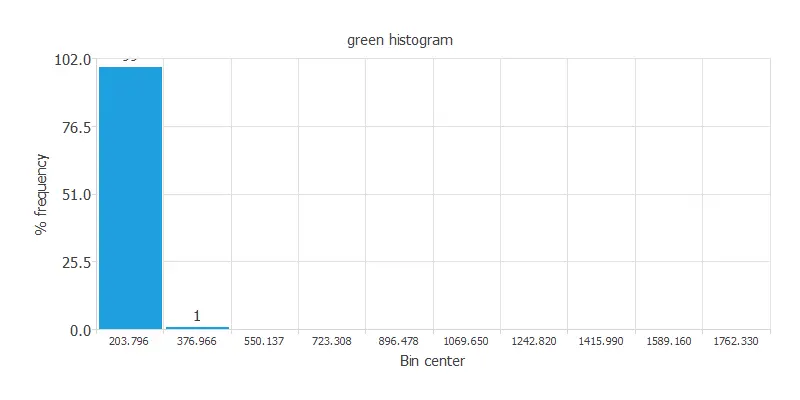As we can see, there are outliers among our data. So first, we must get rid of these instances. The following chart displays the distribution of the green variable after clearing the data of outliers.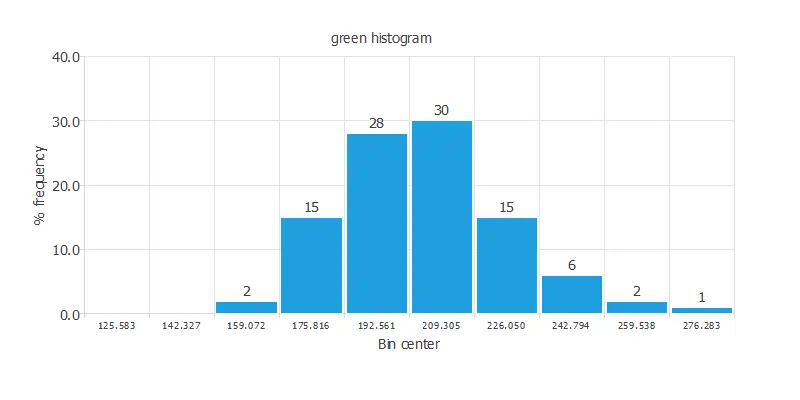As expected, now the distribution of the green variable is correctly displayed. A uniform distribution of the data is always desired. This chart shows a normal distribution of the instances.

## 3. Neural network

Now we have to configure the neural network that represents the classification function.

The number of inputs is 5, and the number of outputs is 1. Therefore, our neural network will be composed of 5 scaling neurons and one probabilistic neuron. We will assume three hidden neurons in the perceptron layer as a first guess.

The binary probabilistic method will be set for this case since here we have a binary classification model. Nevertheless, choosing the continuous probabilistic method would also be correct.

The following picture shows a graph of the neural network for this example.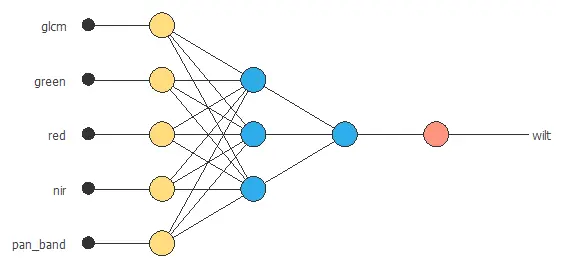## 4. Training strategy

The loss index defines the task that the neural network is required to accomplish. The normalized squared error with strong L2 regularization is used here.

The learning problem is finding a neural network that minimizes the loss index. A neural network fits the data set (error term) without undesired oscillations (regularization term).

The procedure used to carry out the learning process is called an optimization algorithm. The optimization algorithm is applied to the neural network to obtain the minimum possible loss. The type of training is determined by how the neural network's parameters take place.

The quasi-Newton method is used here as the optimization algorithm in the training strategy.

The following chart shows how the training and selection errors decrease with the optimization algorithm epochs during the training process.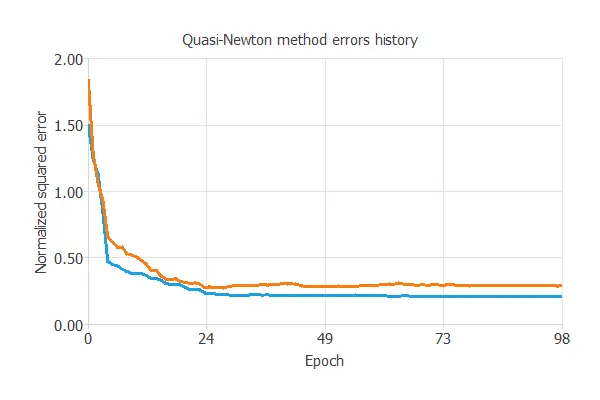The final values are training error = 0.206 NSE and selection error = 0.288 NSE, respectively.

## 5. Model selection

The objective of model selection is to find a network architecture with the best generalization properties, that is, that which minimizes the error on the selected instances of the data set.

More specifically, we want to find a neural network with a selection error of less than 0.288, which is the value that we have achieved so far.

Order selection algorithms train several network architectures with a different number of neurons and select that with the smallest selection error.

The incremental order method starts with a small number of neurons and increases the complexity at each iteration. The following chart shows the training error (blue) and the selection error (orange) as a function of the number of neurons.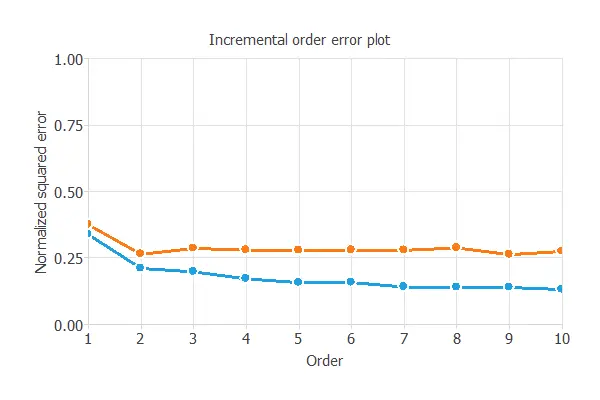After model selection, an optimum selection error of 0.263 NSE has been found for 2 hidden neurons. Below the final network architecture is displayed.## 6. Testing analysis

The last step is to test the generalization performance of the trained neural network.

In the confusion matrix, the rows represent the target classes and the columns the output classes for the testing target data set. The diagonal cells in each table show the number of correctly classified cases, and the off-diagonal cells show the misclassified instances.

The following table shows the confusion elements for this application. The following table contains the elements of the confusion matrix.

Predicted positive Predicted negative
Real positive 42 (37.8%) 5 (4.5%)
Real negative 7 (6.31%) 57 (51.4%)

The next list depicts the binary classification tests for this application:

• Classification accuracy: 89.2% (ratio of correctly classified samples).
• Error rate: 10.8% (ratio of misclassified samples).
• Sensitivity: 89.3% (percentage of actual positive classified as positive).
• Specificity: 89.1% (percentage of actual negative classified as negative).

Therefore, we can state that this data implies a sound performance of the predictive model.

## 7. Model deployment

The neural network is now ready to predict outputs for inputs that it has never seen.

Below, a specific prediction having determined values for the model's input variables is shown.

• glcm: 127.369
• green: 204.672
• red: 105.426
• nir: 447.619
• pan_band: 20.5116

The model predicts that the previous values correspond to a region of diseased trees.

We can also use Response Optimization. The objective of the response optimization algorithm is to exploit the mathematical model to look for optimal operating conditions.
Indeed, the predictive model allows us to simulate different operating scenarios and adjust the control variables to improve efficiency.

An example is to minimize the mean green value while maintaining the diseased probability below the desired value.

The next table resumes the conditions for this problem.

Variable name Condition
GLCM None
Green Minimize
Red None
NIR None
Pan band None
Diseased probability Less than 0.5

The next list shows the optimum values for previous conditions.

• glcm: 155.592.
• green: 127.886.
• red: 143.262.
• nir: 1501.06.
• pan_band: 11.9173.
• diseased_probability: 22%

The mathematical expression, represented by the neural network, which can be exported to any specific software, is written below.

```scaled_glcm = (glcm-127.369)/10.3021;
scaled_green = (green-204.672)/22.6547;
scaled_red = (red-105.426)/23.3449;
scaled_nir = (nir-447.619)/143.758;
scaled_pan_band = (pan_band-20.5116)/6.32208;
y_1_1 = Logistic (2.48167+ (scaled_glcm*-1.12785)+ (scaled_green*-3.77149)+ (scaled_red*1.21979)+ (scaled_nir*1.95294)+ (scaled_pan_band*-0.324738));
y_1_2 = Logistic (-0.649461+ (scaled_glcm*-0.5216)+ (scaled_green*2.05632)+ (scaled_red*-4.68758)+ (scaled_nir*0.950784)+ (scaled_pan_band*-0.377092));
non_probabilistic_wilt = Logistic (-1.9049+ (y_1_1*5.65551)+ (y_1_2*-6.72356));
wilt = binary(non_probabilistic_wilt);

logistic(x){
return 1/(1+exp(-x))
}

binary(x){
if x < decision_threshold
return 0
else
return 1
}

```

## References

• The data for this problem has been taken from the UCI Machine Learning Repository.
• Johnson, B., Tateishi, R., Hoan, N., 2013. A hybrid pansharpening approach and multiscale object-based image analysis for mapping diseased pine and oak trees. International Journal of Remote Sensing, 34 (20), 6969-6982.